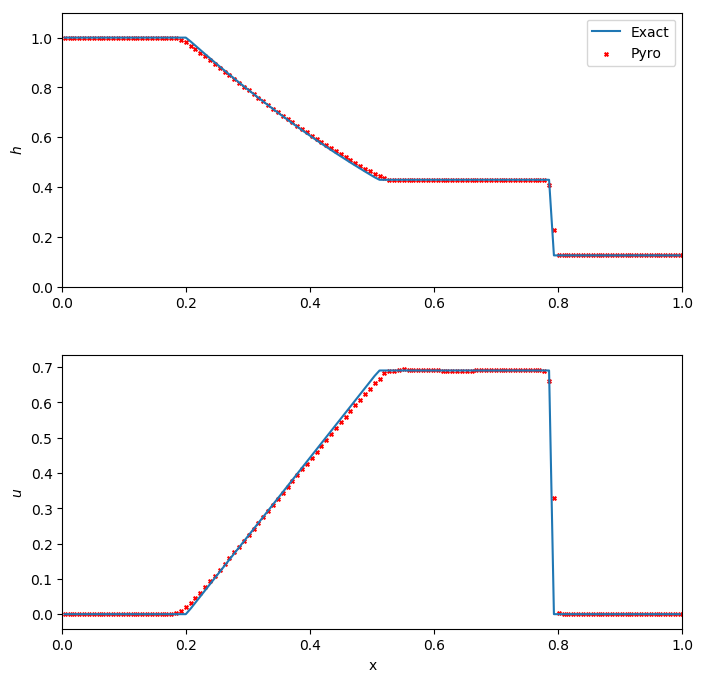# Shallow water solver¶

The (augmented) shallow water equations take the form:

$\begin{split}\frac{\partial h}{\partial t} + \nabla \cdot (h U) &= 0 \\ \frac{\partial (h U)}{\partial t} + \nabla \cdot (h U U) + \frac{1}{2}g\nabla h^2 &= 0 \\ \frac{\partial (h \psi)}{\partial t} + \nabla \cdot (h U \psi) &= 0\end{split}$

with $$h$$ is the fluid height, $$U$$ the fluid velocity, $$g$$ the gravitational acceleration and $$\psi = \psi(x, t)$$ represents some passive scalar.

The implementation here has flattening at shocks and a choice of Riemann solvers.

The main parameters that affect this solver are:

• section: [driver]

option

value

description

cfl

0.8

• section: [particles]

option

value

description

do_particles

0

particle_generator

grid

• section: [swe]

option

value

description

use_flattening

0

apply flattening at shocks (1)

cvisc

0.1

artifical viscosity coefficient

limiter

2

limiter (0 = none, 1 = 2nd order, 2 = 4th order)

grav

1.0

gravitational acceleration (in y-direction)

riemann

Roe

HLLC or Roe

## Example problems¶

### dam¶

The dam break problem is a standard hydrodynamics problem, analagous to the Sod shock tube problem in compressible hydrodynamics. It considers a one-multidimensional problem of two regions of fluid at different heights, initially separated by a dam. The problem then models the evolution of the system when this dam is removed. As for the Sod problem, there exists an exact solution for the dam break probem, so we can check our solution against the exact solutions. See Toro’s shallow water equations book for details on this problem and the exact Riemann solver.

Because it is one-dimensional, we run it in narrow domains in the x- or y-directions. It can be run as:

./pyro.py swe dam inputs.dam.x
./pyro.py swe dam inputs.dam.y


A simple script, dam_compare.py in analysis/ will read a pyro output file and plot the solution over the exact dam break solution (as given by Stoker (1958) and Wu, Huang & Zheng (1999)). Below we see the result for a dam break run with 128 points in the x-direction, and run until t = 0.3 s.We see excellent agreement for all quantities. The shock wave is very steep, as expected. For this problem, the Roe-fix solver performs slightly better than the HLLC solver, with less smearing at the shock and head/tail of the rarefaction.

The quad problem sets up different states in four regions of the domain and watches the complex interfaces that develop as shocks interact. This problem has appeared in several places (and a detailed investigation is online by Pawel Artymowicz). It is run as:

./pyro.py swe quad inputs.quad


### kh¶

The Kelvin-Helmholtz problem models three layers of fluid: two at the top and bottom of the domain travelling in one direction, one in the central part of the domain travelling in the opposite direction. At the interface of the layers, shearing produces the characteristic Kelvin-Helmholtz instabilities, just as is seen in the standard compressible problem. It is run as:

./pyro.py swe kh inputs.kh


## Exercises¶

### Explorations¶

• There are multiple Riemann solvers in the swe algorithm. Run the same problem with the different Riemann solvers and look at the differences. Toro’s shallow water text is a good book to help understand what is happening.

• Run the problems with and without limiting—do you notice any overshoots?

### Extensions¶

• Limit on the characteristic variables instead of the primitive variables. What changes do you see? (the notes show how to implement this change.)

• Add a source term to model a non-flat sea floor (bathymetry).